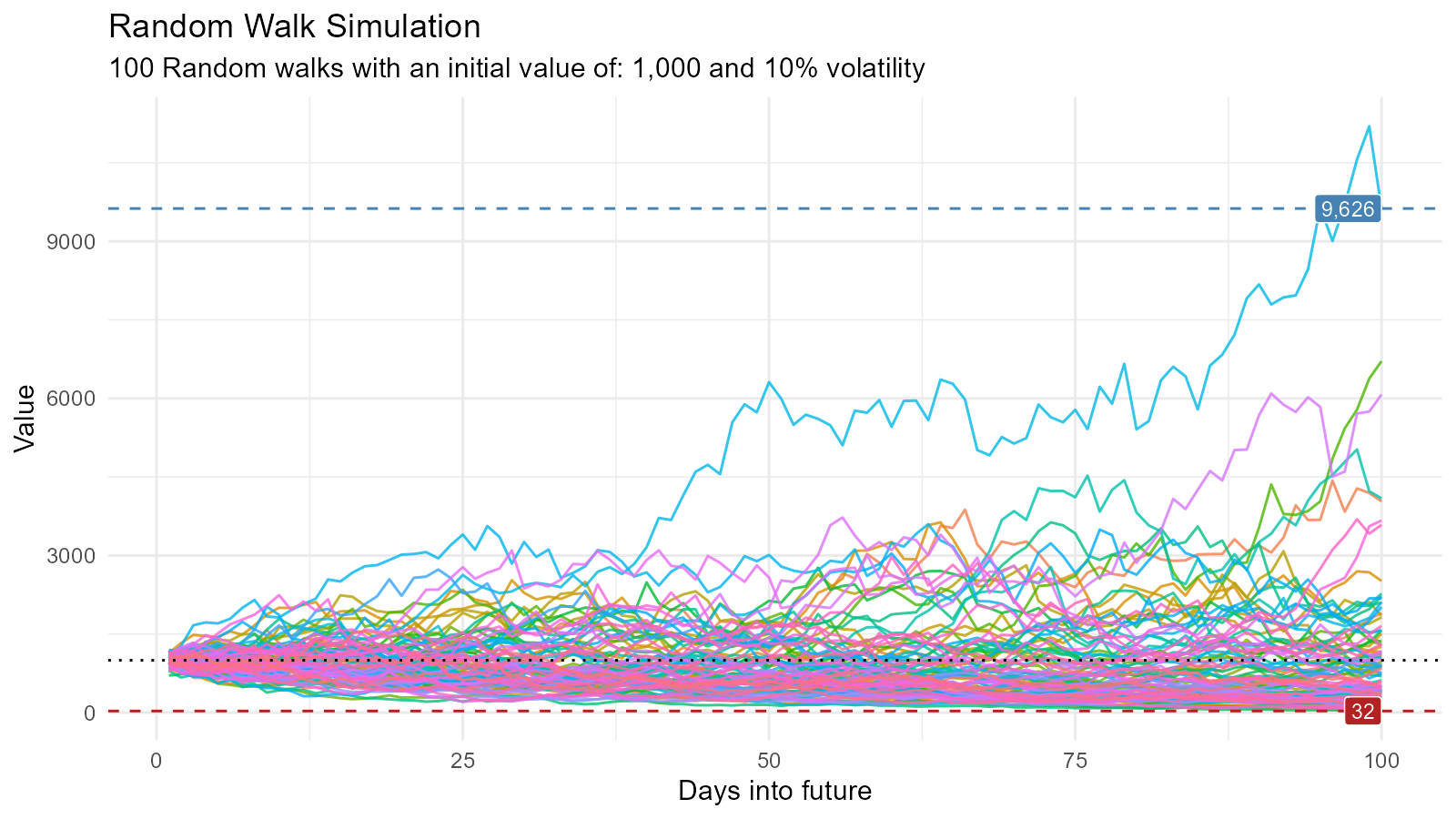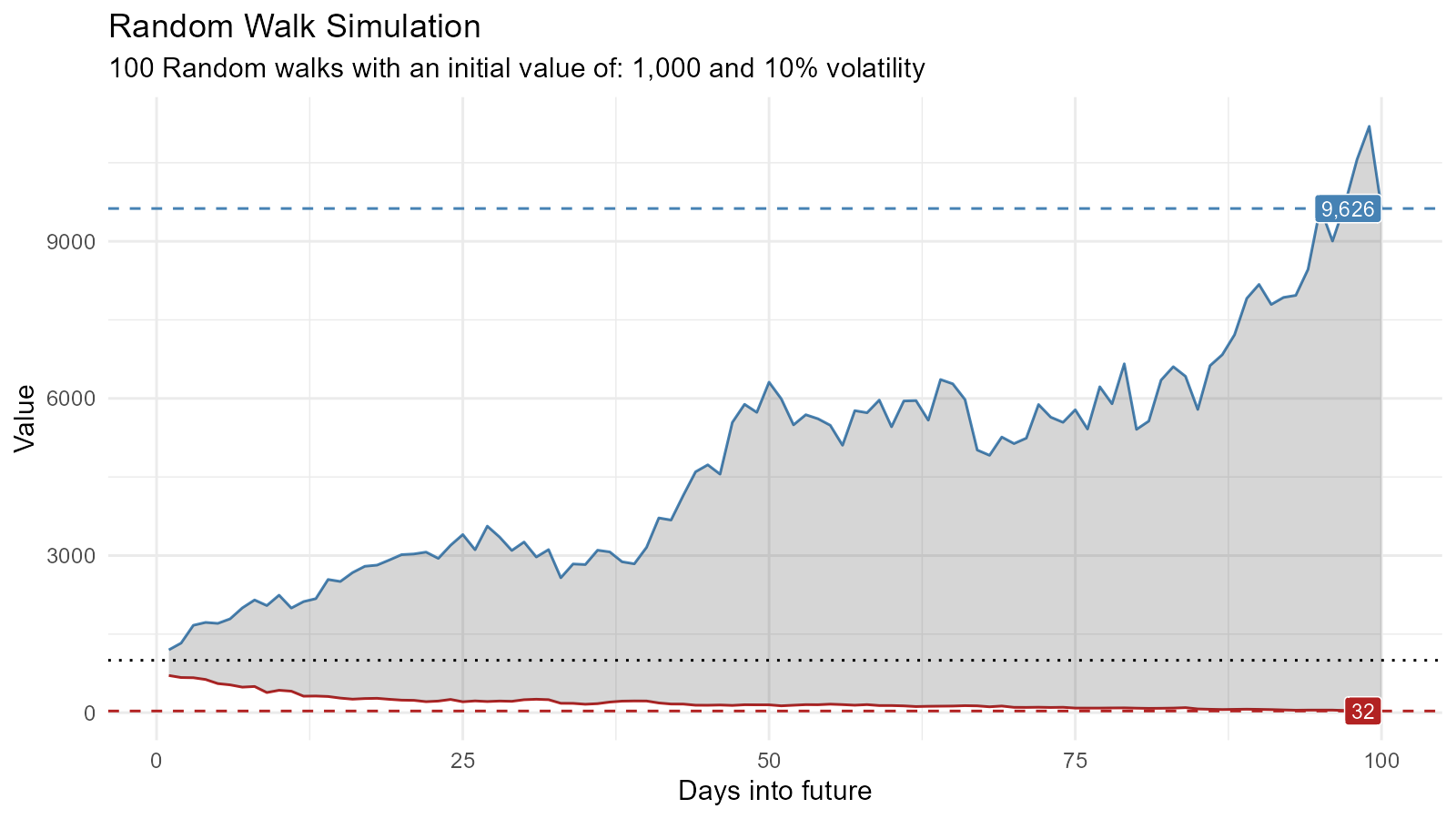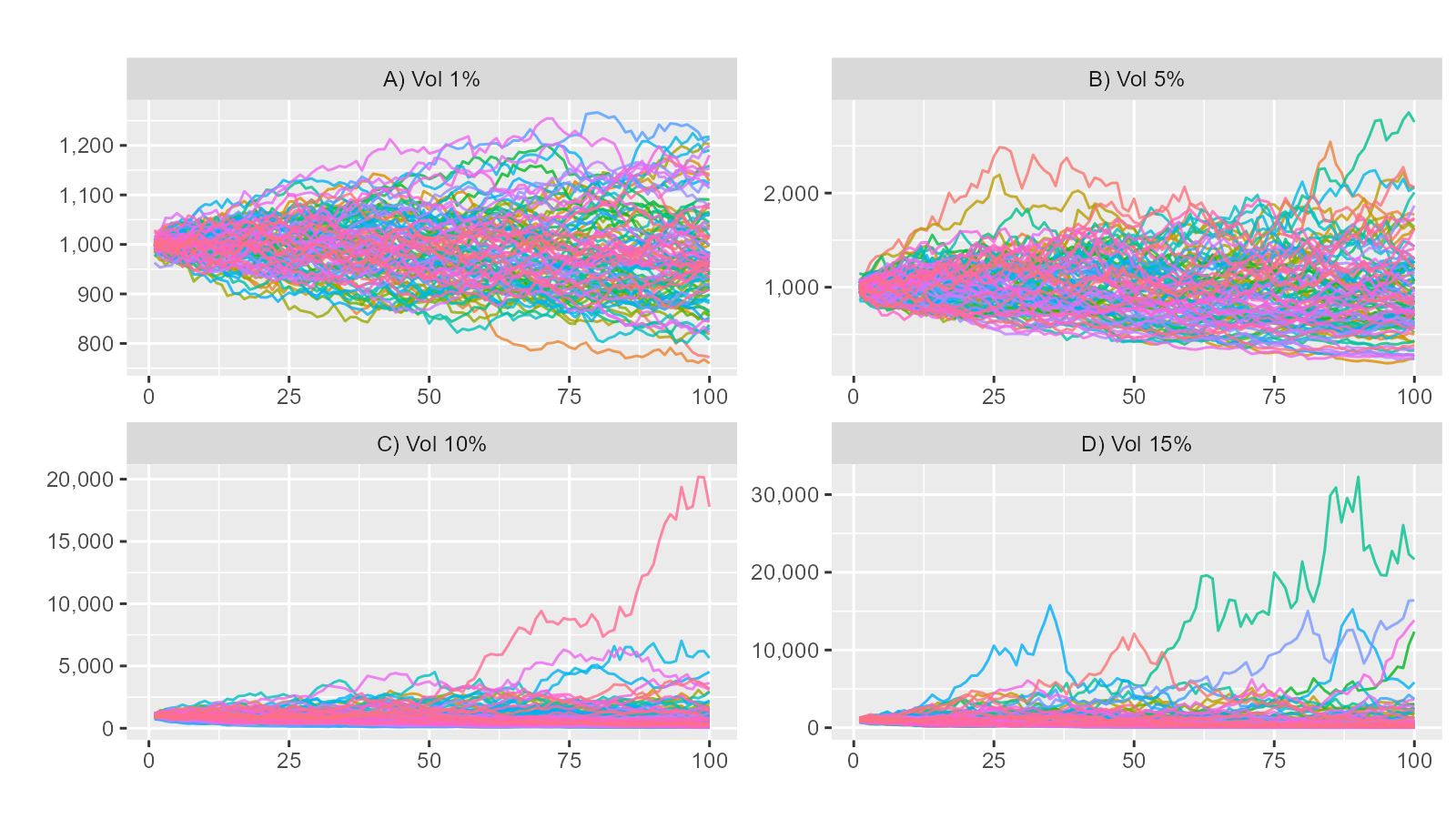library(healthyR.ts)
library(ggplot2)
library(dplyr)

Lets generage data and take a look

df <- ts_random_walk()
#> # A tibble: 6 × 4
#>     run     x         y cum_y
#>   <dbl> <dbl>     <dbl> <dbl>
#> 1     1     1 -0.0416    958.
#> 2     1     2 -0.0899    872.
#> 3     1     3 -0.000568  872.
#> 4     1     4  0.0575    922.
#> 5     1     5 -0.0453    880.
#> 6     1     6 -0.0161    866.
glimpse(df)
#> Rows: 10,000
#> Columns: 4
#> $run <dbl> 1, 1, 1, 1, 1, 1, 1, 1, 1, 1, 1, 1, 1, 1, 1, 1, 1, 1, 1, 1, 1, 1… #>$ x     <dbl> 1, 2, 3, 4, 5, 6, 7, 8, 9, 10, 11, 12, 13, 14, 15, 16, 17, 18, 1…
#> $y <dbl> -0.0416280464, -0.0899276218, -0.0005680194, 0.0574549060, -0.04… #>$ cum_y <dbl> 958.3720, 872.1878, 871.6924, 921.7754, 880.0218, 865.8492, 974.…

Now that the data has been generated, lets take a look at it.

df %>%
ggplot(
mapping = aes(
x = x
, y = cum_y
, color = factor(run)
, group = factor(run)
)
) +
geom_line(alpha = 0.8) +
ts_random_walk_ggplot_layers(df)That is still pretty noisy, so lets see this in a different way. Lets clear this up a bit to make it easier to see the full range of the possible volatility of the random walks.

library(dplyr)
library(ggplot2)

df %>%
group_by(x) %>%
summarise(
min_y = min(cum_y),
max_y = max(cum_y)
) %>%
ggplot(
aes(x = x)
) +
geom_line(aes(y = max_y), color = "steelblue") +
geom_line(aes(y = min_y), color = "firebrick") +
geom_ribbon(aes(ymin = min_y, ymax = max_y), alpha = 0.2) +
ts_random_walk_ggplot_layers(df)Lets look at volatility from several different percentages.


# Random Walk for volatility range 1-15%
df1 <- ts_random_walk(.sd = 0.01)
df2 <- ts_random_walk(.sd = 0.05)
df3 <- ts_random_walk(.sd = 0.10)
df4 <- ts_random_walk(.sd = 0.15)

# Merge data frames into one
df_merged <- dplyr::bind_rows(
df1 %>% mutate(ver = "A) Vol 1%"),
df2 %>% mutate(ver = "B) Vol 5%"),
df3 %>% mutate(ver = "C) Vol 10%"),
df4 %>% mutate(ver = "D) Vol 15%")
)

# Plot range between minimum and maximum values
df_merged %>%
ggplot(aes(
x = x, y = cum_y,
color = factor(run), group = factor(run)
)) +
geom_line(alpha = 0.8) +
labs(title = "", x = "", y = "") +
facet_wrap(~ver, scales = "free") +
scale_y_continuous(labels = scales::comma) +
theme(legend.position = "none")